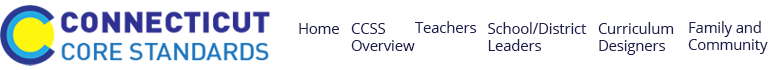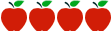### Grade 6: Number System Fluency

Rating:#### Common Core Standards

Content Standards

6.NS.1 - Interpret and compute quotients of fractions, and solve word problems involving division of fractions by fractions, e.g., by using visual fraction models and equations to represent the problem.

6.NS.2 - Fluently divide multi-digit numbers using the standard algorithm.

6.NS.3 - Fluently add, subtract, multiply, and divide multi-digit decimals using the standard algorithm for each operation.

6.NS.4 - Find the greatest common factor of two whole numbers less than or equal to 100 and the least common multiple of two whole numbers less than or equal to 12. Use the distributive property to express a sum of two whole numbers 1-100 with a common factor as a multiple of a sum of two whole numbers with no common factor.

Standards for Mathematical Practice

MP.1  Make sense of problems and persevere in solving them.

MP.2  Reason abstractly and quantitatively.

MP.6  Attend to precision.

MP.7  Look for and make use of structure.

#### Description of Unit

This unit “Number System Fluency” from the Georgia DOE is a collection of 9 tasks that encompass computation with positive rational numbers.  Explicit instruction and assessment are absent.  In order for this document to be effectively used, lesson plans would have to be developed around each of the 9 activities/applications. The unit centers on the concepts of number system computation with positive rational numbers and builds the foundational knowledge leading to work with negative rational numbers. It builds on learning from previous work in grades 4 and 5 to reach fluency in computation with positive rational numbers.

#### Cautions

Connecticut teachers should be aware that the unit does not include the following components:

• Structures for implementing and completing performance tasks. There are no instructional notes (answer keys, rubrics, student work samples).
• differentiation or supports for students working above/below grade level, English language learners, or students with disabilities.
• use of technology or media.

#### Rationale for Selection

• The rigorous tasks encourage contextual understanding of the standards.
• There are relevant, thought-provoking tasks for students to complete.
• The tasks might be beneficial for formative assessment of key concepts of the unit.
• The tasks are rooted in applications that help develop student thinking skills in problem-solving scenarios.